Courses

# Examples of cables Mechanical Engineering Notes | EduRev

## Mechanical Engineering : Examples of cables Mechanical Engineering Notes | EduRev

The document Examples of cables Mechanical Engineering Notes | EduRev is a part of the Mechanical Engineering Course Engineering Mechanics - Notes, Videos, MCQs & PPTs.
All you need of Mechanical Engineering at this link: Mechanical Engineering

Example 31.1

Determine reaction components at A and B, tension in the cable and the sag yB, and yE  of the cable shown in Fig. 31.4a. Neglect the self weight of the cable in the analysis.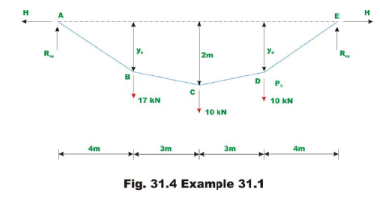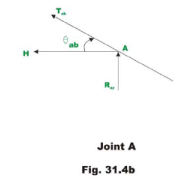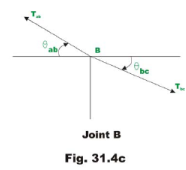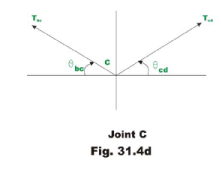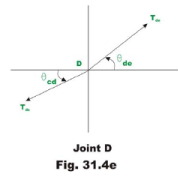Since there are no horizontal loads, horizontal reactions at A and B should be the same. Taking moment about E, yields

Ray *14 −17 * 20 −10 * 7 − 10 * 4 = 0

Rαy = 280/14 =20kN      Rey = 37 20 17kN.

Now horizontal reaction H may be evaluated taking moment about point  of all forces left of C .

Ray *7 − H * 2−17*3 = 0

H = 44.5 kN

Taking moment about B of all the forces left of B and setting MB= 0 , we get

Ray H * yB =0;   yB = 80/44.50 = 1.798 m

Similarly, yD = 68/44.50 =1.528m

To determine the tension in the cable in the segment AB , consider the equilibrium of joint A (vide Fig.31.4b).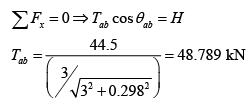The tension Tab may also be obtained as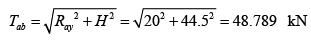Now considering equilibrium of joint B,C and D one could calculate tension in different segments of the cable.

Segment bc

Applying equations of equilibrium,

∑Fx = 0 ⇒ Tab cos θab = Tbc cosθbc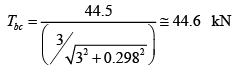See Fig.31.4c

Segment cd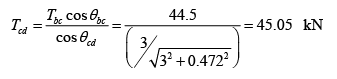See Fig.31.4d.

See Fig.31.4e.

Segment de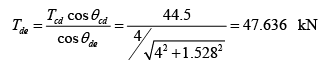The tension Tde may also be obtained as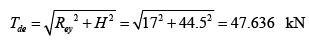Example 31.2

A cable of uniform cross section is used to span a distance of 40m as shown in Fig 31.5. The cable is subjected to uniformly distributed load of 10 kN/m. run. The left support is below the right support by 2 m and the lowest point on the cable  is located below left support by 1 m. Evaluate the reactions and the maximum and minimum values of tension in the cable.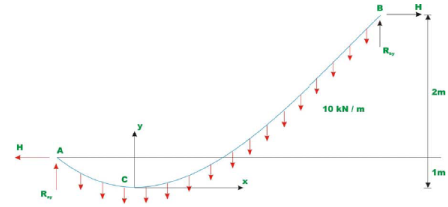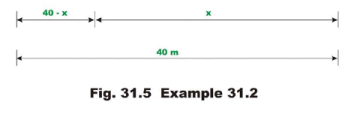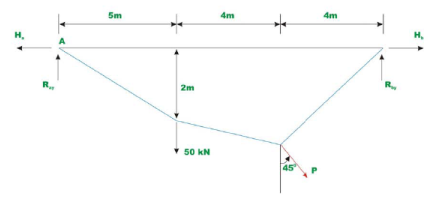Fig. 31.6 Example 31.3

Assume the lowest point C to be at distance of x m from B . Let us place our origin of the co-ordinate system xy at C . Using equation 31.5, one could write,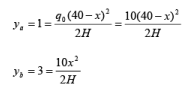(1)    (2)

where ya and yb be the y co-ordinates of supports A and B respectively. From equations 1 and 2, one could evaluate the value of x . Thus,

10(4x - x2) = 10x2/3 ⇒ x =25.359 m

From equation 2, the horizontal reaction can be determined.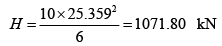Now taking moment about A of all the forces acting on the cable, yields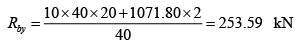Writing equation of moment equilibrium at point B , yields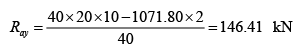Tension in the cable at supports A and B are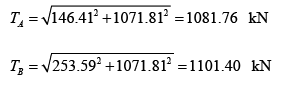The tension in the cable is maximum where the slope is maximum as T cosθ = H . The maximum cable tension occurs at B and the minimum cable tension occurs at C where dy/dx = θ = 0 and Tc = H = 1071.81 kN

Example 31.3

A cable of uniform cross section is used to support the loading shown in Fig 31.6. Determine the reactions at two supports and the unknown sag yC.

Taking moment of all the forces about support B ,

Ray =1/10 [350 + 300 +100yc]             (1)

Ray = 65 +10 yc

Taking moment about B of all the forces left of B and settingMB=0 , we get,

Ray*3-Ha*2 =0

⇒ Ha= 1.5Ray            (2)

Taking moment about C of all the forces left of C and setting = 0 , we get

∑Mc = 0   Ray * 7-Ha * yc -50*4 =0

Substituting the value of in terms of in the above equation,

7Ray-1.5Ray yc -200 =0       (3)

Using equation (1), the above equation may be written as,

yc2+1.833yc -17 =0       (4)

Solving the above quadratic equation, can be evaluated. Hence,

yC = 3.307m.

Substituting the value of  in equation (1),

Ray = 98.07 kN

From equation (2),

Ha =1.5Ray = 147.05 kN

Now the vertical reaction atD,Rdy calculated by taking moment of all the forces about A,

Rdy * 10 − 100 * 7 + 100 * 3.307 − 50 * 3 = 0

Rdy =51.93  kN.

Taking moment of all the forces right of C about C , and noting that ∑Mc =0,

Rdy 3 = Hd* yc⇒Hd=47.109  kN.

Offer running on EduRev: Apply code STAYHOME200 to get INR 200 off on our premium plan EduRev Infinity!

## Engineering Mechanics - Notes, Videos, MCQs & PPTs

30 videos|72 docs|65 tests

,

,

,

,

,

,

,

,

,

,

,

,

,

,

,

,

,

,

,

,

,

;